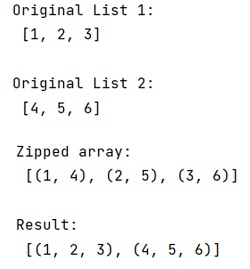# What is the inverse function of zip?

Learn about the inverse function of zip function in Python?
Submitted by Pranit Sharma, on December 21, 2022

NumPy is an abbreviated form of Numerical Python. It is used for different types of scientific operations in python. Numpy is a vast library in python which is used for almost every kind of scientific or mathematical operation. It is itself an array which is a collection of various methods and functions for processing the arrays.

## Inverse function of zip

Python zip() method is used to return a single object, having mapped values from all the containers. It takes some sort of iterables or containers and it is used to map the similar index of multiple containers so that they can be used just using a single entity.

Here, suppose we have used the zip() function from the NumPy library to sort tuples and now we have a list containing all the tuples. We have since modified that list and now we need to restore the tuples so we can use our data.

For this purpose, we need to use the (*) operator with the zip() function result to reverse this result so that if the zip() method gives us zipped_data, *zipped_data given the inverse if zip().

Let us understand with the help of an example,

## Python code to demonstrate the example of inverse function of zip

```# Import numpy
import numpy as np

# Creating two lists
a = [1,2,3]
b = [4,5,6]

# Display original lists
print("Original List 1:\n",a,"\n")
print("Original List 2:\n",b,"\n")

# Using zip
Zipped = zip(a,b)

# Display zipped data
print("Zipped data:\n",Zipped,"\n")

# Unzipping
res = zip(*Zipped)

# Display result
print("Result:\n",res)
```

Output: